Ex 13.1

Chapter 13 Class 9 Surface Areas and Volumes
Serial order wise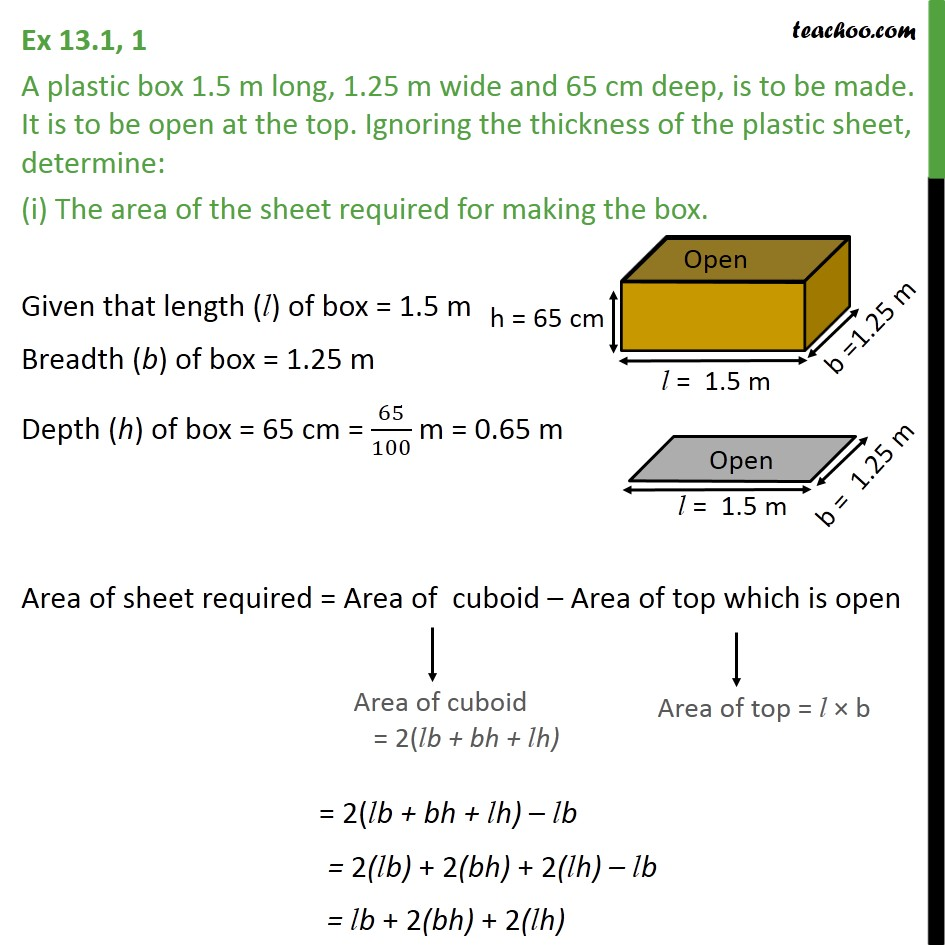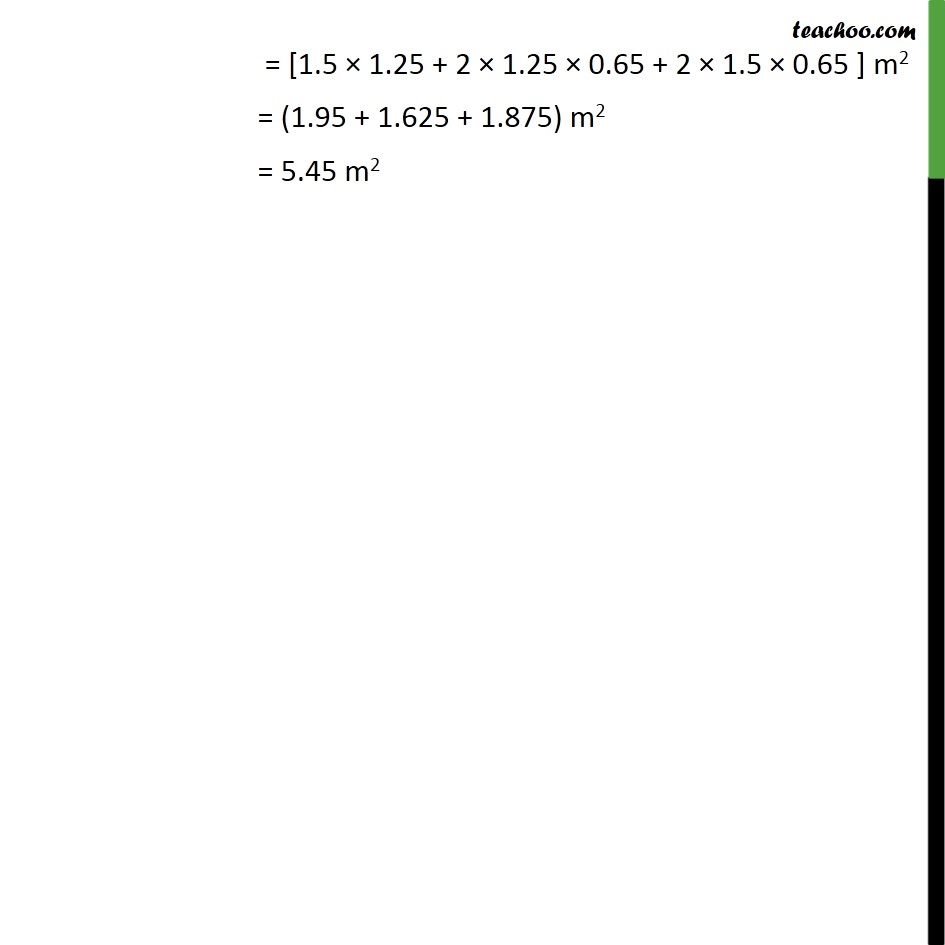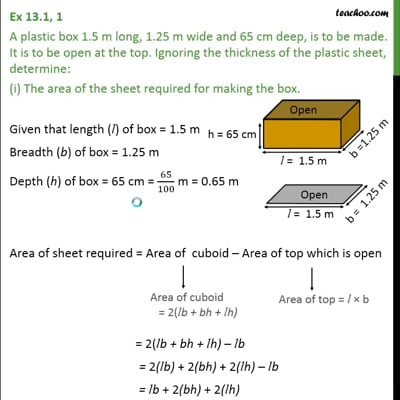This video is only available for Teachoo black users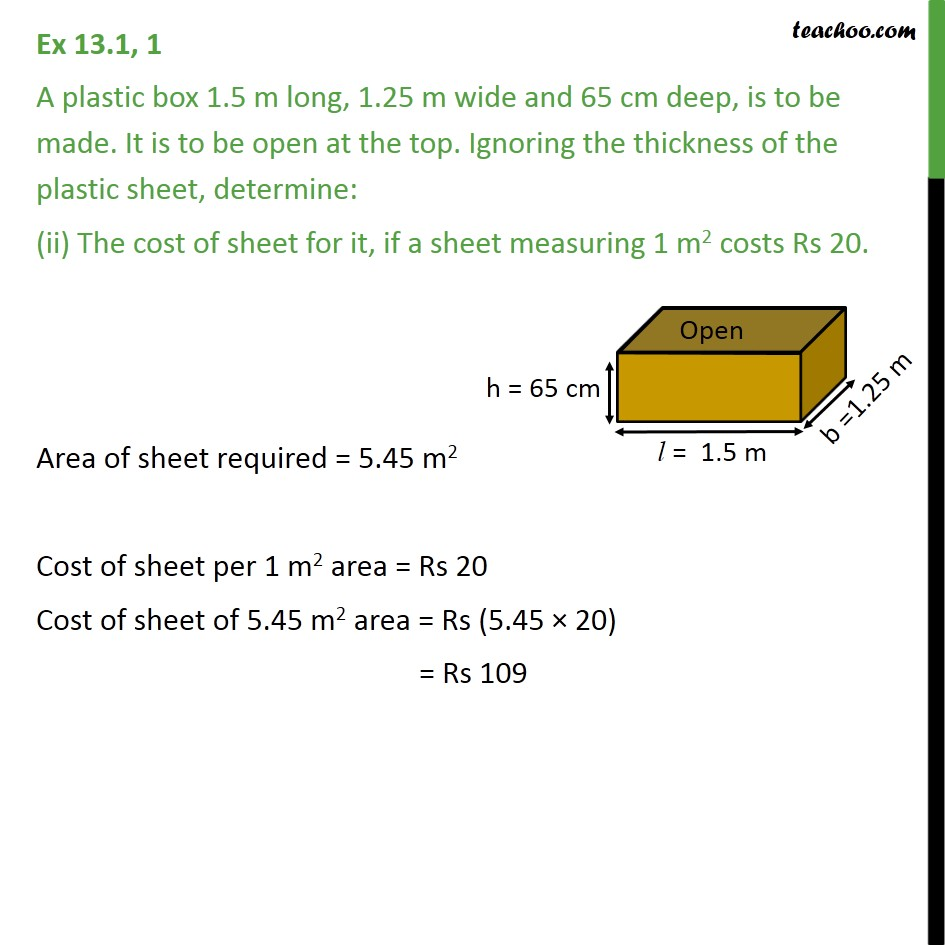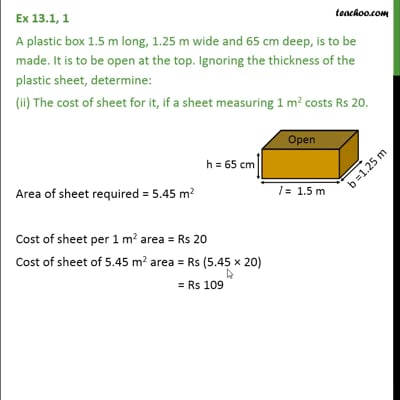This video is only available for Teachoo black users

Solve all your doubts with Teachoo Black (new monthly pack available now!)

### Transcript

Ex 13.1, 1 A plastic box 1.5 m long, 1.25 m wide and 65 cm deep, is to be made. It is to be open at the top. Ignoring the thickness of the plastic sheet, determine: (i) The area of the sheet required for making the box. Given that length (l) of box = 1.5 m Breadth (b) of box = 1.25 m Depth (h) of box = 65 cm = 65/100 m = 0.65 m Area of sheet required = Area of cuboid Area of top which is open = 2(lb + bh + lh) lb = 2(lb) + 2(bh) + 2(lh) lb = lb + 2(bh) + 2(lh) = [1.5 1.25 + 2 1.25 0.65 + 2 1.5 0.65 ] m2 = (1.95 + 1.625 + 1.875) m2 = 5.45 m2 Ex 13.1, 1 A plastic box 1.5 m long, 1.25 m wide and 65 cm deep, is to be made. It is to be open at the top. Ignoring the thickness of the plastic sheet, determine: (ii) The cost of sheet for it, if a sheet measuring 1 m2 costs Rs 20. Area of sheet required = 5.45 m2 Cost of sheet per 1 m2 area = Rs 20 Cost of sheet of 5.45 m2 area = Rs (5.45 20) = Rs 109